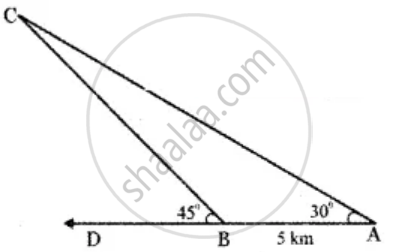Tamil Nadu Board of Secondary EducationHSC Arts Class 11th

# Two soldiers A and B in two different underground bunkers on a straight road, spot an intruder at the top of a hill. The angle of elevation of the intruder from A and B to the ground level in the - Mathematics

Sum

Two soldiers A and B in two different underground bunkers on a straight road, spot an intruder at the top of a hill. The angle of elevation of the intruder from A and B to the ground level in the eastern direction are 30° and 45° respectively. If A and B stand 5km apart, find the distance of the intruder from B

#### Solution

Let A and B be the two positions of the soldiers.

AC – direction of the intruder seen from A.

BC – the direction of the intruder seen from B.

∠BAC = 30° angle of elevation of the intruder from A.

∠PBC = 45° angle of elevation of the intruder from B.Distance between A and B = 5k.m.

In ∆ABC,

∠ABC = 180° – 45° = 135°

∠BCA = 180° – (135° + 30°)

= 180° – 165°

= 15°

Using sine formula

"BC"/(sin∠"BAC") = "AB"/(sin∠"ACB")

"BC"/(sin 30^circ) = 5/(sin 15^circ)

"BC"/(1/2) = 5/(sin(45^circ - 30^circ))

2BC = 5/(sin 45^circ cos 30^circ - cos 45^circ sin 30^circ)

2BC = 5/(1/sqrt(2) * sqrt(3)/2 - 1/sqrt(2) * 1/2)

2BC = 5/((sqrt(3) - 1)/(2sqrt(2))

= (2sqrt(2) xx 5)/(sqrt(3) - 1)

BC = (5sqrt(2))/(sqrt(3) - 1) k.m.

Distance of intruder from B = (5sqrt(2))/(sqrt(3) - 1) k.m.

Concept: Application to Triangle
Is there an error in this question or solution?

Share